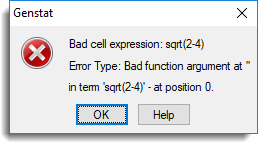1. Home
2. Cell Calculations

# Cell Calculations

A single value calculation can be entered into a numerical cell in the spreadsheet by starting the calculation with an equals sign (=), and then following with a calculation using standard Genstat syntax. These calculations can also be entered in the edit spreadsheet cell dialog opened by double-clicking a cell or by using the F8 key.

These calculations are not stored, and are lost once the result has been calculated. These are only provided to enable quick in-cell calculations and the calculations menu should be used for other column calculations. Cell-wise calculations are considered to be both a major strength and weaknesses of any spreadsheet. You have the flexibility to perform easy calculation but can easily end up with inconsistent formulae within a column.

The length of the cell calculation is limited by the character buffer size of the column, for example, 45 characters for numerical columns. To enter formulae that are greater than 45 characters you can use the Edit Spreadsheet Cell dialog.

## Functions

Not all Genstat functions are supported, but in general, functions that give scalar results with scalar input are supported. The list of functions supported are:
abs, sqrt, sin, cos, tan, arcsin, arccos, arctan, sinh, cosh, tanh, exp, log, log10, factorial, ncombinations, int, modulo, radians, degrees, logit, alogit, date and constants.

Note the arguments of date are date(day,month,year).

These functions can be abbreviated to 4 letters, with the exception of constants, which can be abbreviated to c. Function arguments are separated by semicolons (;).

## Operators

The operators supported are ** (power), * (multiplication), / (division), + and . Parentheses can be used to change the order of operations. Numbers can also be entered using the e notation for powers of 10 (i.e. 7e+5 = 700000, 7e-5 = 0.00007). The Boolean operators ==, /= = are not supported.

## Note

The constants pi (3.14159265…) and e (2.71828182…) can be entered using the constants function: c(‘pi’) and c(‘e’)

## Errors

On an illegal calculation (e.g. log(0) or sqrt(-1), 1/0, misspelled function or syntax error), a dialog will appear which lists the fault and the term that it occurs in. You can either correct the calculation, and then retry it, or use the escape key (Esc) to abort the calculation.

## Example

The cell calculation can just be typed into any numerical cell:Pressing return results in the calculation being replaced by its result:The following are some cell calculations that could be used:
=2*(3+5)/(7-9)
=ncomb(10;5)*(2**3)+5
=date(17;10;2005)-date(4;12;1959)
=sqrt(2-4) (This contains an error, giving the error dialogs):Note: This error dialog only occurs for domain errors in functions.## Acknowledgements

The cell calculator is based on a code provided by Mark Morley, Victoria, Canada (morley@camosun.bc.ca).